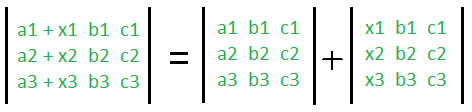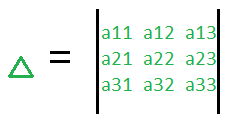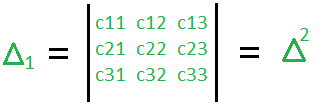# Properties of Determinants of Matrices

Determinant of a Matrix is a scalar property of that Matrix. Determinant is a special number that is defined for only square matrices (plural for matrix). Square matrix have same number of rows and columns.

Determinant is used to know whether the matrix can be inverted or not, it is useful in analysis and solution of simultaneous linear equations (Cramer’s rule), used in calculus, used to find area of triangles (if coordinates are given) and more. Determinant of a matrix A is denoted by |A| or det(A).

Properties of Determinants of Matrices:

1. Determinant evaluated across any row or column is same.
2. If all the elements of a row (or column) are zeros, then the value of the determinant is zero.
3. Determinant of a Identity matrix () is 1.
4. If rows and columns are interchanged then value of determinant remains same (value does not change). Therefore, det(A) = det(), hereis transpose of matrix A.
5. If any two row (or two column) of a determinant are interchanged the value of the determinant is multiplied by -1.
6. If all elements of a row (or column) of a determinant are multiplied by some scalar number k, the value of the new determinant is k times of the given determinant. Therefore, If A be an n-rowed square matrix and K be any scalar. Then |KA| =|A| .
7. If two rows (or columns) of a determinant are identical the value of the determinant is zero.
8. Let A and B be two matrix, then det(AB) = det(A)*det(B).
9. If A be a matrix then, || =.
10. Determinant of Inverse of matrix can be defined as || =.
11. Determinant of diagonal matrix, triangular matrix (upper triangular or lower triangular matrix) is product of element of the principle diagonal.
12. In a determinant each element in any row (or column) consists of the sum of two terms, then the determinant can be expressed as sum of two determinants of same order. For example,13. If B is obtained by adding c-times a row of A to a different row, the.
14. Let A be a matrix then,
|adj(A)| =|adj(adj(A))| =,

15. If value of determinantbecomes zero by substituting x =, then x-is a factor of.
16. Here, cij denotes the cofactor of elements of aij in.17. In a determinant the sum of the product of the elements of any row (or column) with the cofactors of the corresponding elements of any other row (or column) is zero. For example,
d = ai1*Aj1 + ai2*Aj2 + ai3*Aj3 +…… + ain*Ajn, here Aj1, Aj2, Aj3 …Ajn are cofactors along elements of jth row.

18. Letare the Eigenvalues of A (square matrix of order n). Then det(A) =, product of Eigenvalues.

Attention reader! Don’t stop learning now. Get hold of all the important DSA concepts with the DSA Self Paced Course at a student-friendly price and become industry ready.

My Personal Notes arrow_drop_upCheck out this Author's contributed articles.

If you like GeeksforGeeks and would like to contribute, you can also write an article using contribute.geeksforgeeks.org or mail your article to contribute@geeksforgeeks.org. See your article appearing on the GeeksforGeeks main page and help other Geeks.

Please Improve this article if you find anything incorrect by clicking on the "Improve Article" button below.

Article Tags :

1

Please write to us at contribute@geeksforgeeks.org to report any issue with the above content.